# What is the capacitor and the capacitance

## capacitor

A capacitor is a component from electrical engineering for storing electrical energy or electrical charge. You can find more information about the capacitor in the article below.First of all: All those who do not yet know what current, voltage and resistance is or who do not yet know Ohm's law should read the following articles first. Otherwise it will be difficult to follow the rest of the article.

Show:

### What is a capacitor?

A capacitor is a component used in electrical engineering. In this electrical charge or electrical energy can be stored. A capacitor consists of two conductive layers that are separated from one another by an insulator. The so-called plate capacitor is a simple design of the capacitor. It consists of a pair of metal plates that are isolated from one another and each connected to a pole of a voltage source. If you draw an electronic circuit, the circuit symbol of the capacitor looks like this: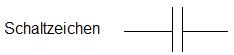What do you need a capacitor for?

Now, of course, the question still arises: What do you need a capacitor for? Here are some areas of application (which, however, will largely exceed the knowledge of electrical engineering in schools):

• As an energy store to supply a consumer with energy. Example: A battery needs to be changed on a system. In order to bridge the voltage failure during the change, a previously charged capacitor is discharged during the change.
• As reactances or frequency-dependent resistances. This knowledge helps when setting up analog filters to dampen interfering frequency components.
• Special designs of capacitors can be used as sensors, which can be used to monitor processes and systems.
Show:

### Calculate capacitor with formulas

We have already learned that a capacitor can store energy. Now, of course, the question arises: how much charge a corresponding capacitor can hold. Because of this, the physical size farad - F for short - introduced.
Notice: The capacity of a capacitor indicates how much electrical charge a capacitor can store at a voltage of 1 volt.

Earlier in the article, we already referred to basic articles on charge, current, voltage, resistance, etc. If you don't know these terms yet, please read them up. Because with these the following formulaic relationships are to be understood: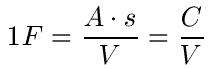Two more formulas follow to calculate charge (Q), current (I), time (t) and voltage (U):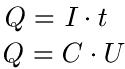Formula plate capacitor

In the school's physics class, teachers like to calculate the capacitance of a plate capacitor. For this reason we would like to take a closer look at the corresponding formula. Important: The formula only applies to the plate capacitor. Other formulas must also be used for other designs.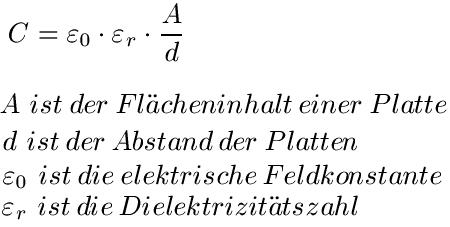Remarks:

• ε0 is a constant with ε0 = 8.854 · 10-12 As / Vm
• εR. depends on the medium (air, paper, glass). The value is taken from tables.

### Plate capacitor example:

We want to calculate the capacitance of a plate capacitor. This has an area of ​​0.0001m2, the plate distance is 0.2mm. The medium is air (εR. = 1).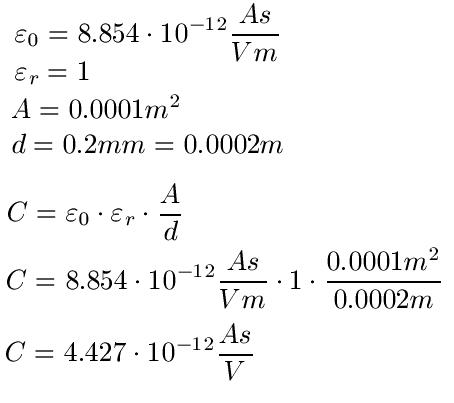Left:

### Who's Online

We have 253 guests online